# Lions Weekly: September 29, 2021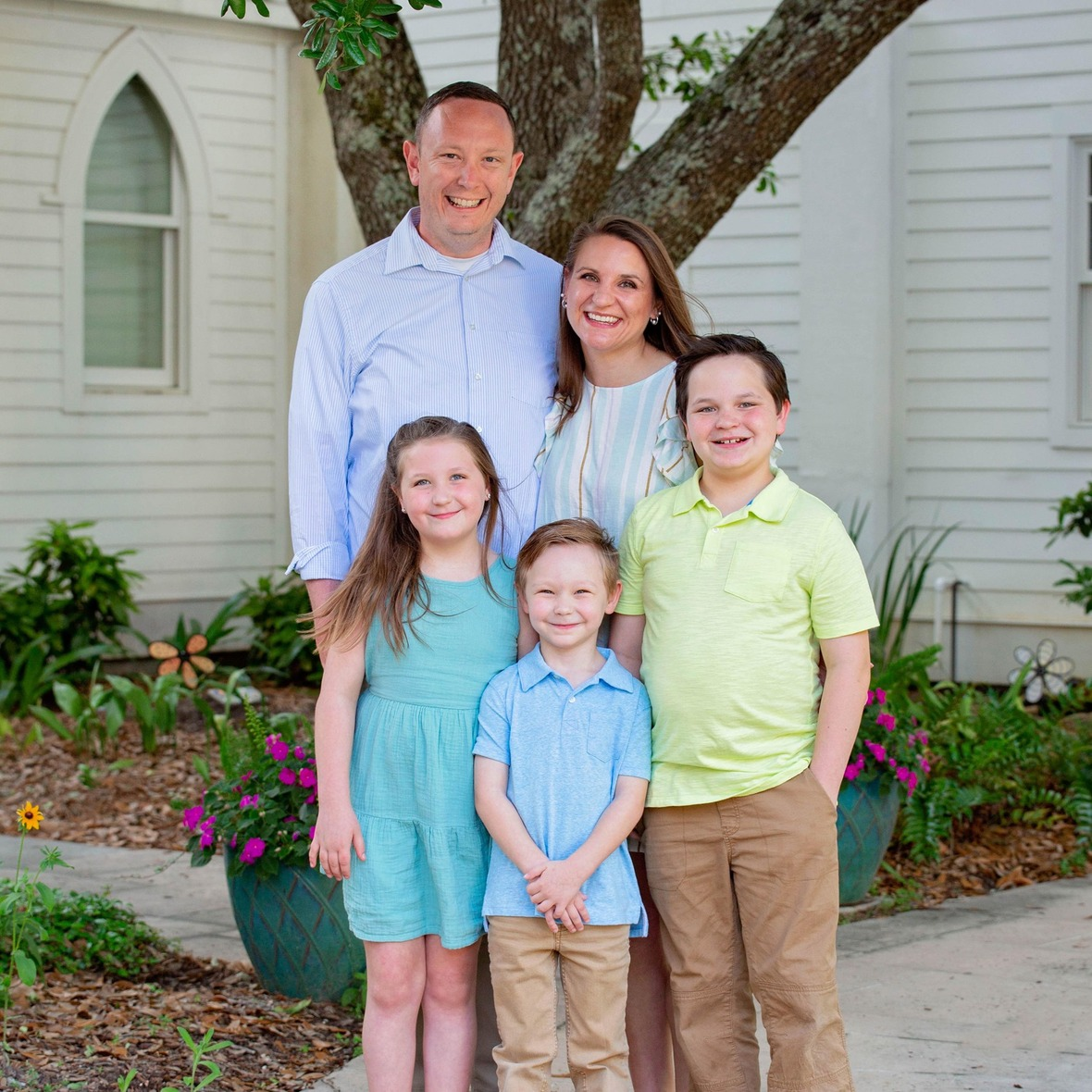# A Note From Mr. Sandefur

Lion Family,

I want to encourage our families to continue to take precautions as we are seeing an increasing number of cases in Covid-19 in our area and within our school community. We are continuing to work to mitigate the spread of the virus while on campus. However, we must remain vigilant even though our numbers have been very low throughout the year thus far.

As we prepare to finish our first academic quarter with excellence, I am reminded of our task as an educational institution to best meet the needs of our students. In our efforts to continually evaluate all that we do, our faculty and staff are looking at ways that we can improve. It has become more evident over the past months across our nation that the educational landscape has shifted dramatically throughout the nationwide pandemic.

Educators are looking at ways to combat learning deficits, the effects of excessive absence from the classroom, as well as the mental and emotional health challenges associated with growing up in a time of such change and instability. Our desire here at TKA is to address all of these concerns while adhering to a biblical worldview that allows our students to see purpose in chaos. As we continue to evaluate curriculum, schedules, activities, and more to meet the needs of our students, I ask that you join with us in prayer for discernment, wisdom, and direction. It is the desire of my heart that we reach the individual needs of our students and families while doing so with the love of Christ on a daily basis.

For those who are interested in learning more about the issues facing education today, I recommend this article as it provides insight into what we are studying to determine the next steps as professional educators: click here

Finally, I want to encourage all members of our TKA family to attend this Friday’s home game (second of the season due to cancellations) as we celebrate our students' gifts and talents. We will be honoring teams from our spring season, cheering our Lions on to victory in a very important region matchup, and honoring those who are battling breast cancer and those who fought the fight so honorably. We encourage everyone who comes to the game to wear pink in honor of Breast Cancer Awareness. You won’t want to miss this opportunity to celebrate with our school family!

Go Lions!

In Christ Alone,

Jeremy Sandefur
President

 table div table+table+table+table div table{width:100%;padding:0}table div table+table+table+table div table img{width:96.23%;padding:0;float:none}table div table+table+table+table div table td{width:100%;padding:0 1.88% 18px}/* styles */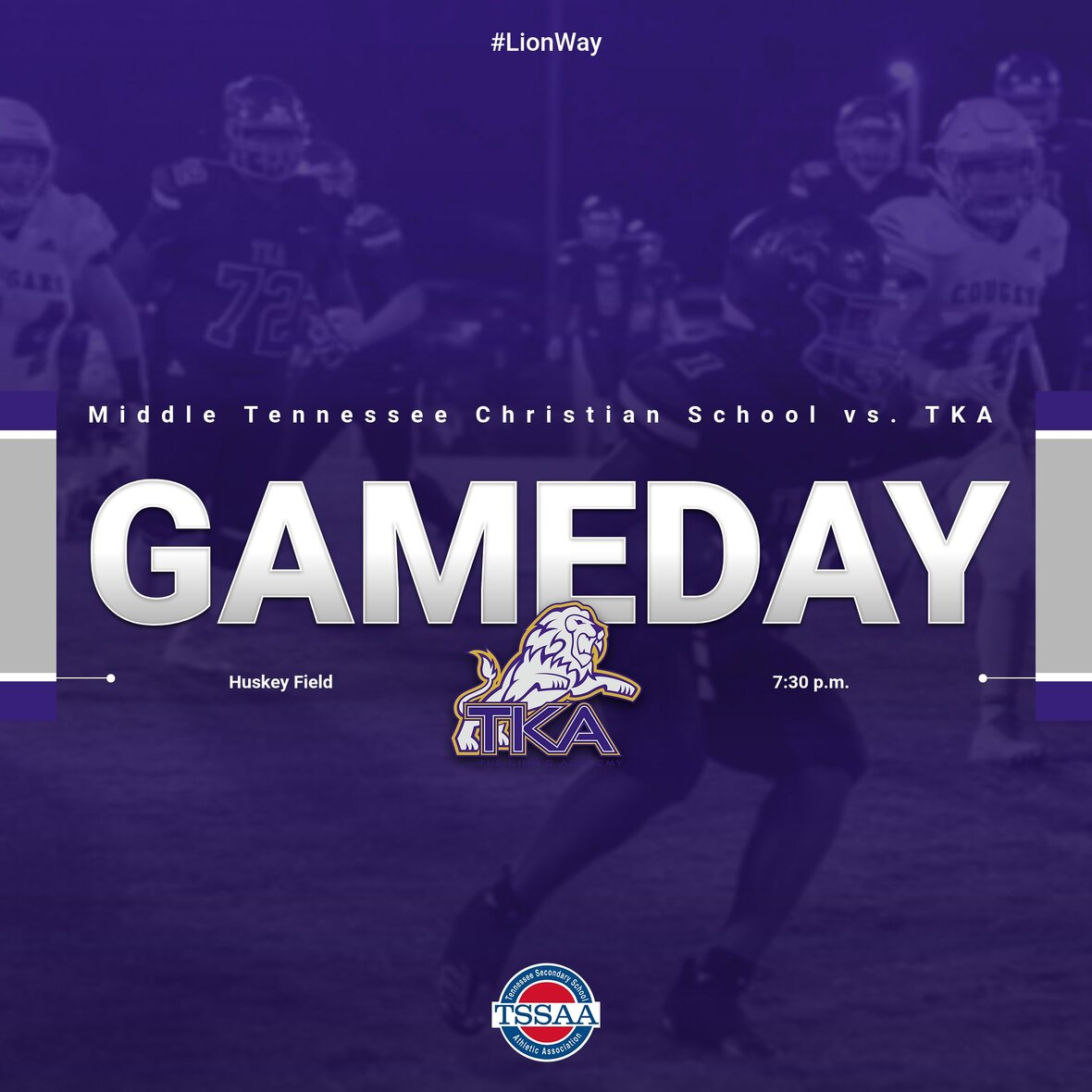# Varsity Football

TKA is excited to host Middle Tennessee Christian this Friday, October 1, in our final regular season home game of 2021. This is a huge game for our football program as they battle to remain in first-place in the East Region. Please click here to purchase tickets via GoFan. Adult tickets are \$8 and student tickets are \$5. Tickets will NOT be sold at the gate, so please be sure to purchase tickets prior to arriving at the game.

Pre-game activities begin at 6:00 p.m. with tailgating followed by the Senior Night Presentation at 7:00 p.m. Kickoff is set for 7:30 p.m. We hope to see everyone at Huskey Field on Friday!

# Tailgating

What: TKA Tailgate!
When: Friday, October 1st. at 6:00 p.m.
Where: Grassy area at stone wall at Huskey Field

Come join us for some fun, games & fellowship before cheering on our Lions football! Kick off at 7:30! Tickets sold separate and in advance!

Hosted by PTF and Team 15:7. PTF will provide cookies (while they last!) for the event!

Bring food and drink for your family! Bring chairs and any yard games! Don’t forget your plates, cups, napkins!

Come hang out, enjoy fellowship, then cheer on our Lions!

This invite is school wide! We want all our Lions included!

 /* styles */
 table div table+table+table+table+table+table+table+table div table{width:100%;padding:0}table div table+table+table+table+table+table+table+table div table img{width:96.23%;padding:0;float:none}table div table+table+table+table+table+table+table+table div table td{width:100%;padding:0 1.88% 18px}/* styles */# Pink Out Day

This Friday, October 1, is pink out day! Students can dress in pink attire.
Remember, dress out day expectations are as follows:
▪ Dress code length (shorts/skirts, etc.) is expected
▪ Dress code footwear is expected
▪ Jeans are allowed (no rips, tears, etc.)
▪ No inappropriate, vulgar, or any print that goes against the mission and vision of TKA is allowed
▪ Hats are allowed but are to be removed in the classroom if asked to allow all students visual access to presented materials, etc.

# Breast Cancer Awareness

In honor of Breast Cancer Awareness Month, we will be having a Pink Out Day on Friday, October 1, throughout the school day and at the football game. During halftime, we will recognize breast cancer survivors with their families on the football field, and honor those families who have lost a loved one due to breast cancer. If you would like to honor someone during halftime, please fill out this form.

On Saturday, October 2, at 10:00 a.m. a breast cancer walk will take place on the campus of TKA. This walk is to honor those who have fought and are continuing to fight breast cancer. We will also be collecting money for someone currently undergoing breast cancer treatments. Please join us as we celebrate with breast cancer survivors, their families and their support teams. We will also honor loved ones lost to breast cancer. There will be some food, music, and pictures will be taken. Masks are encouraged but not required for this event. To sign up for this event, click here.

If you have any questions about either event, please call Jennifer Ayers at (865) 202-8989.

 table div table+table+table+table+table+table+table+table+table+table div table{width:100%;padding:0}table div table+table+table+table+table+table+table+table+table+table div table img{width:96.23%;padding:0;float:none}table div table+table+table+table+table+table+table+table+table+table div table td{width:100%;padding:0 1.88% 18px}/* styles */# Elementary R.O.A.R. t-shirt

We are excited to offer our 2nd annual ROAR Elementary t-shirt. These shirts may be worn on Fridays with the approved dress code.

The front of the shirt will include our school lion logo with our elementary theme R.O.A.R. (Radical Love for Jesus and Others; Opportunities to serve; Show Christ-like Attitude; Speak with Respectful Language). On the back, we will have the year 2021 along with the scripture Galatians 5:22-23. Inside the year, parents can sponsor to have their child’s signature printed.

The annual shirt will provide students a keepsake with each year they attended elementary school along with the opportunity to see the growth of their signature over the years.

The ROAR fundraiser helps us continue to provide resources and support for our teachers and staff in the elementary.

 table div table+table+table+table+table+table+table+table+table+table+table+table div table{width:100%;padding:0}table div table+table+table+table+table+table+table+table+table+table+table+table div table img{width:96.23%;padding:0;float:none}table div table+table+table+table+table+table+table+table+table+table+table+table div table td{width:100%;padding:0 1.88% 18px}/* styles */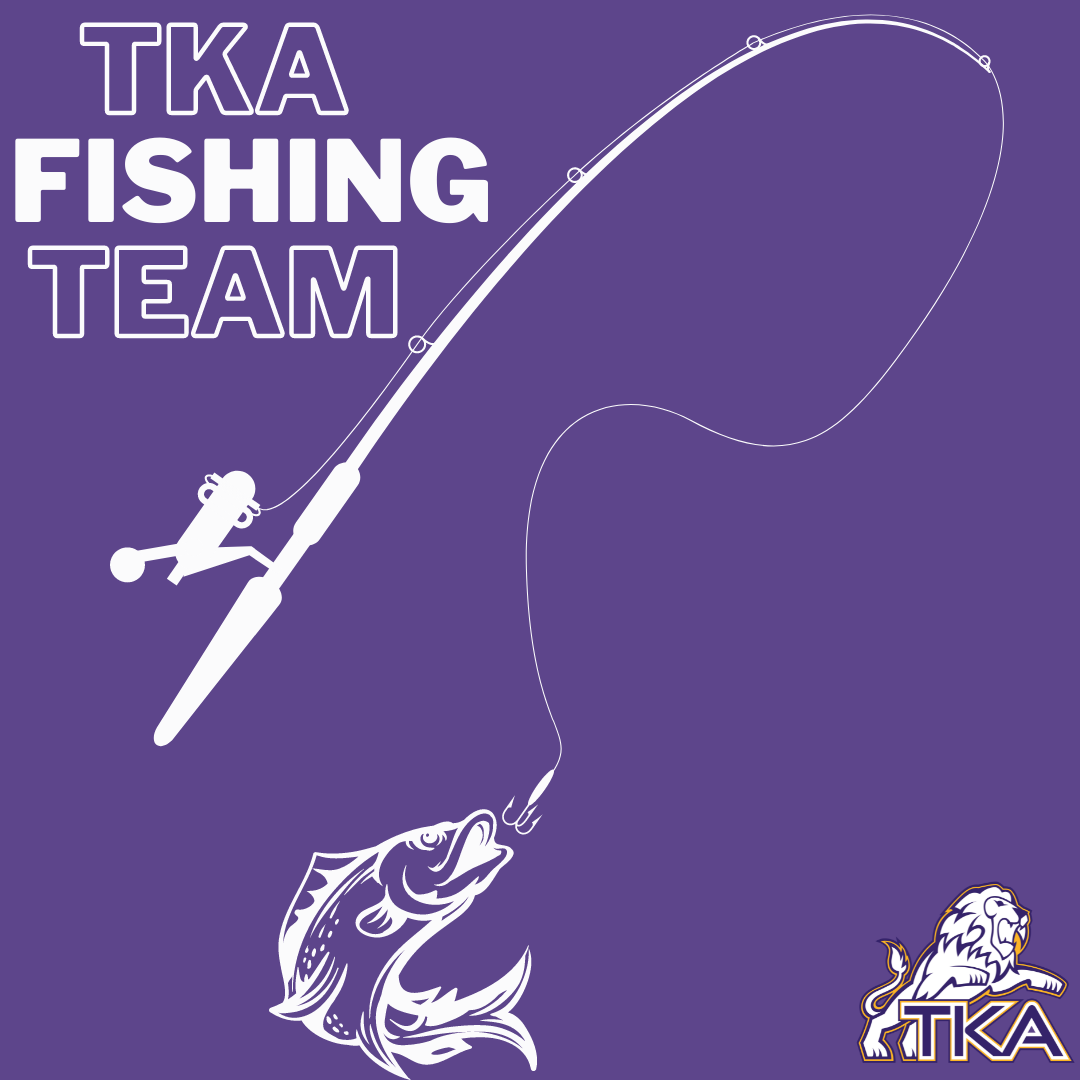# Fishing Team

This Thursday, September 30, our Fishing Team will have a meeting at 6:00 p.m. in Tibbetts.

 table div table+table+table+table+table+table+table+table+table+table+table+table+table+table div table{width:100%;padding:0}table div table+table+table+table+table+table+table+table+table+table+table+table+table+table div table img{width:96.23%;padding:0;float:none}table div table+table+table+table+table+table+table+table+table+table+table+table+table+table div table td{width:100%;padding:0 1.88% 18px}/* styles */# National Honor Society

NHS will host its annual induction ceremony at 6:00 p.m. on Monday, October 4, in Woody Auditorium. All members are encouraged to attend to support inductees. Family and guests are welcome at this event. Contact Mr. Smith (jsmith@thekingsacademy.net) with any questions.

 table div table+table+table+table+table+table+table+table+table+table+table+table+table+table+table+table div table{width:100%;padding:0}table div table+table+table+table+table+table+table+table+table+table+table+table+table+table+table+table div table img{width:96.23%;padding:0;float:none}table div table+table+table+table+table+table+table+table+table+table+table+table+table+table+table+table div table td{width:100%;padding:0 1.88% 18px}/* styles */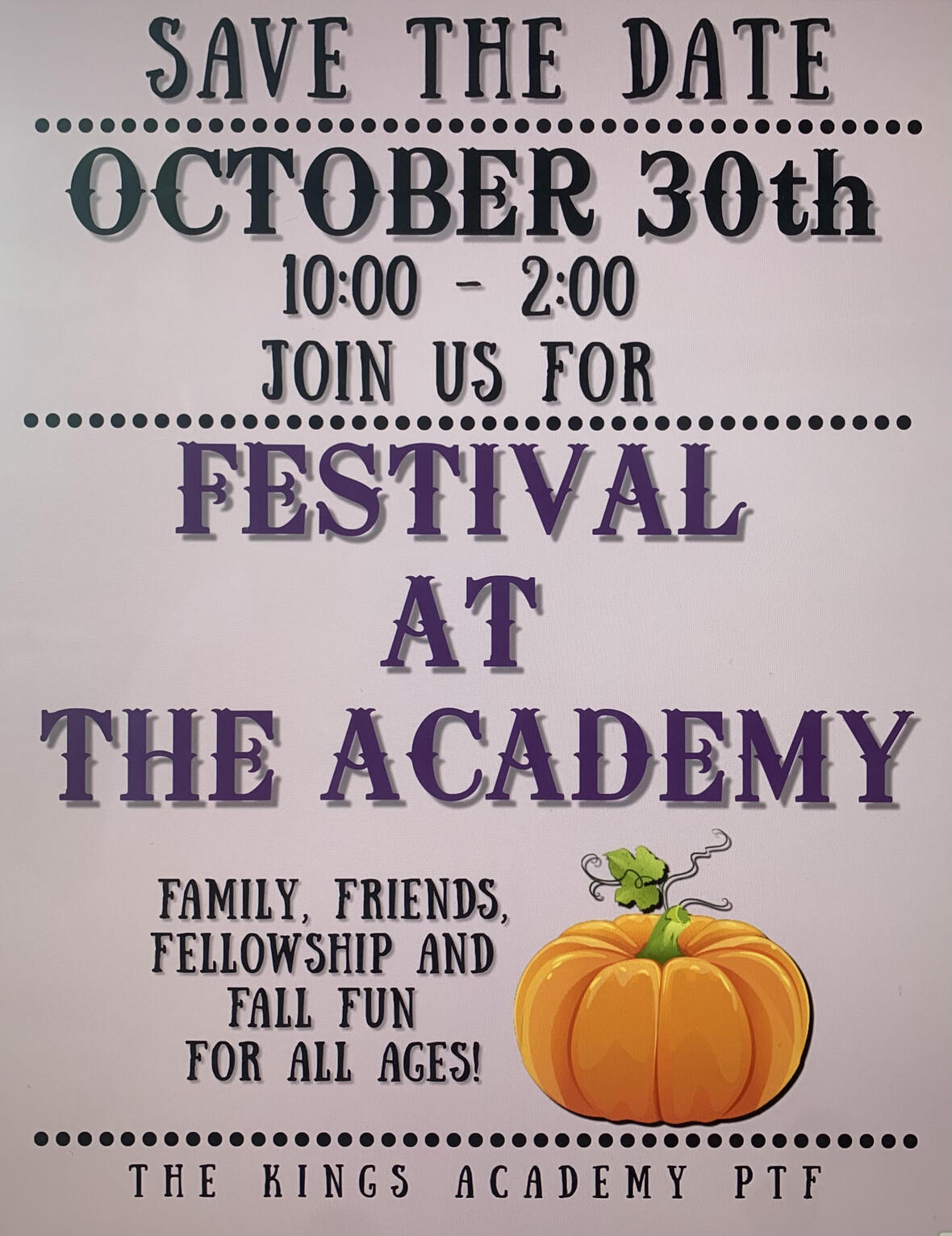Mark your calendars and save the date! The Festival at the Academy is taking place on Saturday, October 30, from 10 a.m. to 2 p.m.

The committee is currently taking donations for the silent auction baskets. If you or your company would like to donate a tax deductible basket, reach out to one of the contacts listed below!

## Contacts for Festival at The Academy:

The King’s Academy (865) 573-8321 ext.5
Kara Pritchard (865) 924-4333
Cherish McLin (865) 604-8303

 table div table+table+table+table+table+table+table+table+table+table+table+table+table+table+table+table+table+table div table{width:100%;padding:0}table div table+table+table+table+table+table+table+table+table+table+table+table+table+table+table+table+table+table div table img{width:96.23%;padding:0;float:none}table div table+table+table+table+table+table+table+table+table+table+table+table+table+table+table+table+table+table div table td{width:100%;padding:0 1.88% 18px}/* styles */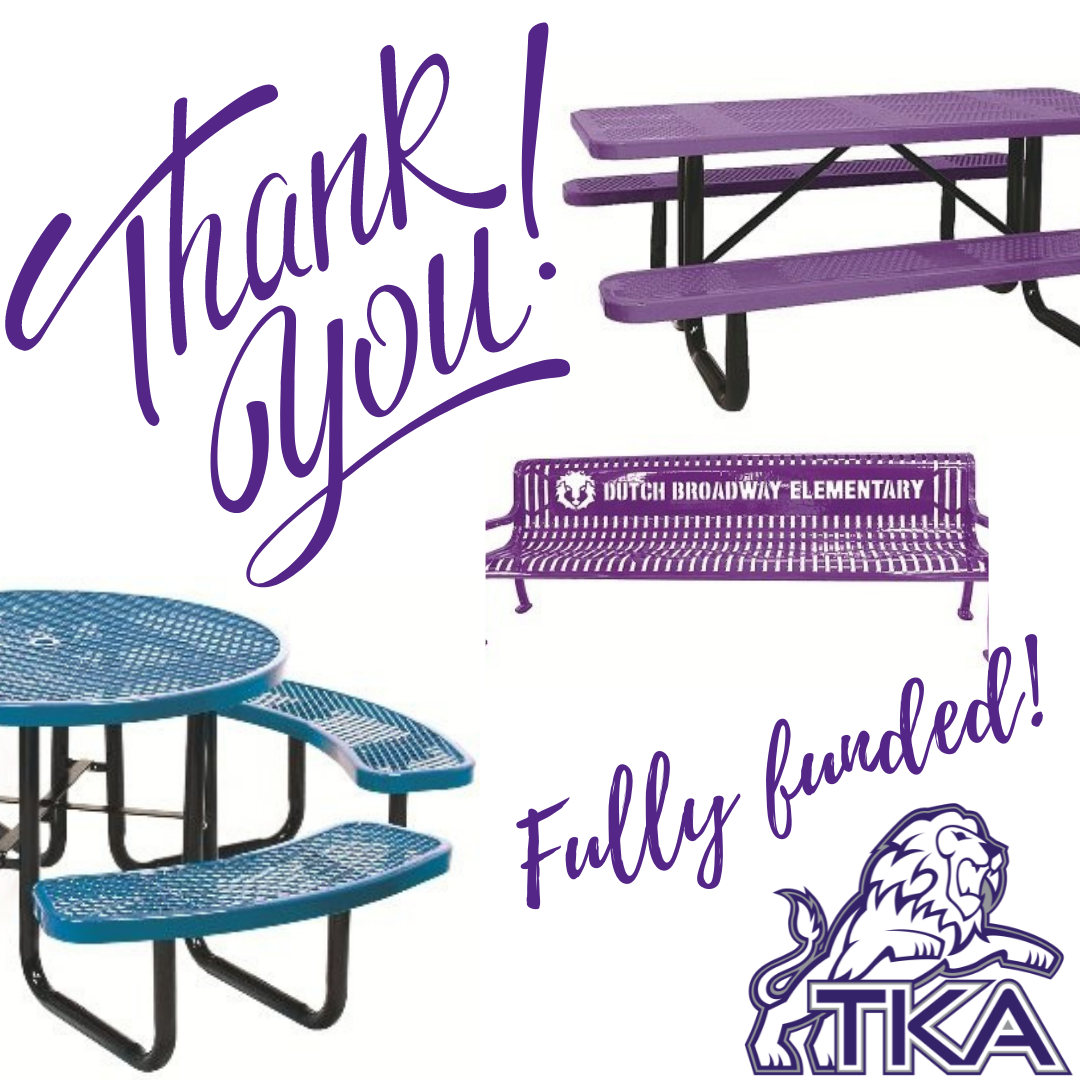# Dr. Carroll Reece Reagan Pavilion

The Dr. Carroll Reece Reagan Pavilion is nearing completion (aiming for the next few weeks), and the benches and tables for the Pavilion are fully funded! Thank you to all families, individuals, and companies who have given so graciously to help make this happen!

 table div table+table+table+table+table+table+table+table+table+table+table+table+table+table+table+table+table+table+table+table div table{width:100%;padding:0}table div table+table+table+table+table+table+table+table+table+table+table+table+table+table+table+table+table+table+table+table div table img{width:96.23%;padding:0;float:none}table div table+table+table+table+table+table+table+table+table+table+table+table+table+table+table+table+table+table+table+table div table td{width:100%;padding:0 1.88% 18px}/* styles */# PreK and Elementary Mulch

A special thank you to MK Equipment Sales, Tri Star Land Management, and PTF for donating seven bags of mulch to help fill our PreK and Elementary playground areas! Our Little Lions are excited about this improvement!table div table+table+table+table+table+table+table+table+table+table+table+table+table+table+table+table+table+table+table+table+table+table+table div table{width:100%;padding:0}table div table+table+table+table+table+table+table+table+table+table+table+table+table+table+table+table+table+table+table+table+table+table+table div table img{width:96.23%;padding:0;float:none}table div table+table+table+table+table+table+table+table+table+table+table+table+table+table+table+table+table+table+table+table+table+table+table div table td{width:100%;padding:0 1.88% 18px}/* styles */# Andrew Seaton Memorial

In memory of TKA parent, Andrew Seaton, the PTF members donated a magnolia tree to be planted in his honor. We are thankful for Mr. Seaton and the legacy he left behind. Please join us as we continue to pray for the Seaton family.

 table div table+table+table+table+table+table+table+table+table+table+table+table+table+table+table+table+table+table+table+table+table+table+table+table+table div table{width:100%;padding:0}table div table+table+table+table+table+table+table+table+table+table+table+table+table+table+table+table+table+table+table+table+table+table+table+table+table div table img{width:96.23%;padding:0;float:none}table div table+table+table+table+table+table+table+table+table+table+table+table+table+table+table+table+table+table+table+table+table+table+table+table+table div table td{width:100%;padding:0 1.88% 18px}/* styles */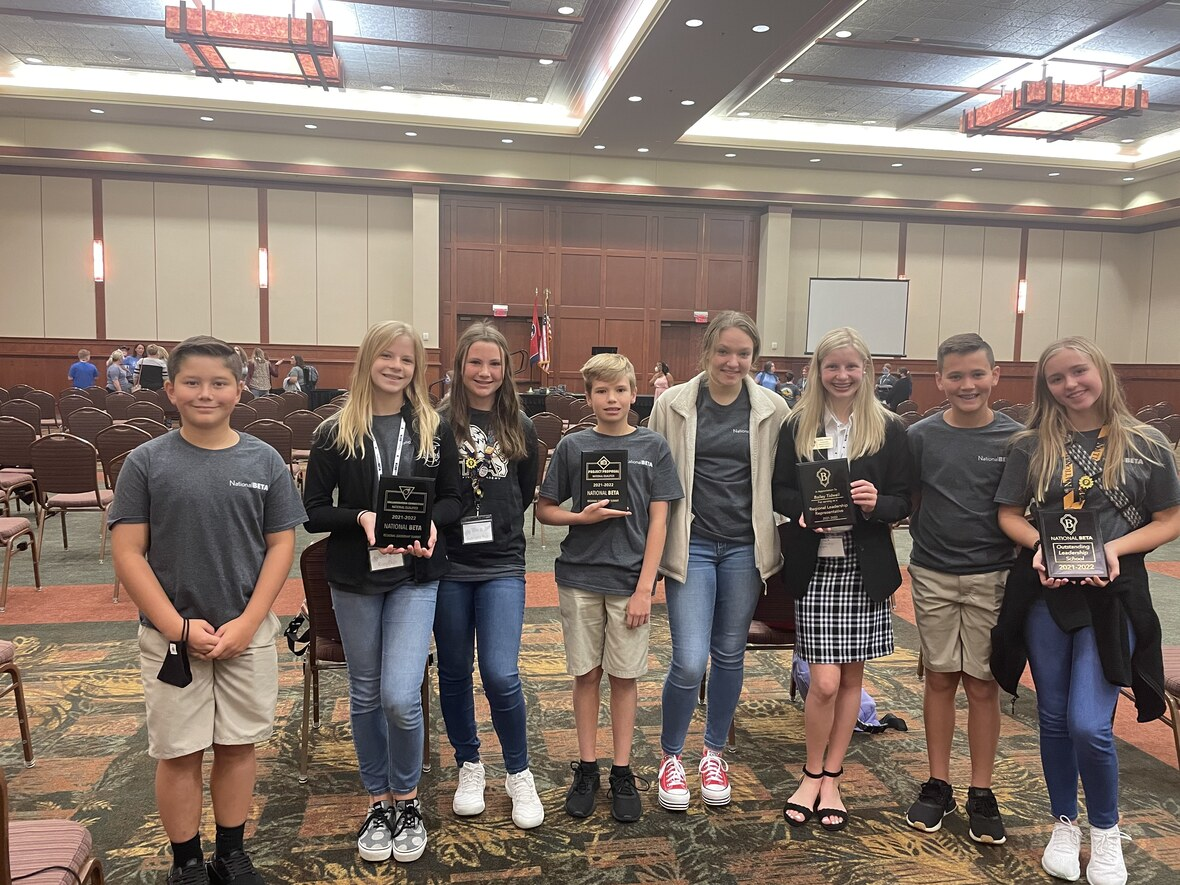# Jr. Beta Shines

Our Jr. Beta officers and 7th grade reps have competed at the Leadership Summit at the Wilderness. They participated as a club in the following competitions: Lead Outside The Box, Project Proposal and Collaboration Connection. They did such an awesome job and qualified for Nationals in every competition! Bailey Tidwell has served as a Regional Leadership Representative at the Summit, and she was recognized for the fantastic job she did. We are so very proud of our Lions for representing TKA at the Summit!

 table div table+table+table+table+table+table+table+table+table+table+table+table+table+table+table+table+table+table+table+table+table+table+table+table+table+table+table div table{width:100%;padding:0}table div table+table+table+table+table+table+table+table+table+table+table+table+table+table+table+table+table+table+table+table+table+table+table+table+table+table+table div table img{width:96.23%;padding:0;float:none}table div table+table+table+table+table+table+table+table+table+table+table+table+table+table+table+table+table+table+table+table+table+table+table+table+table+table+table div table td{width:100%;padding:0 1.88% 18px}/* styles */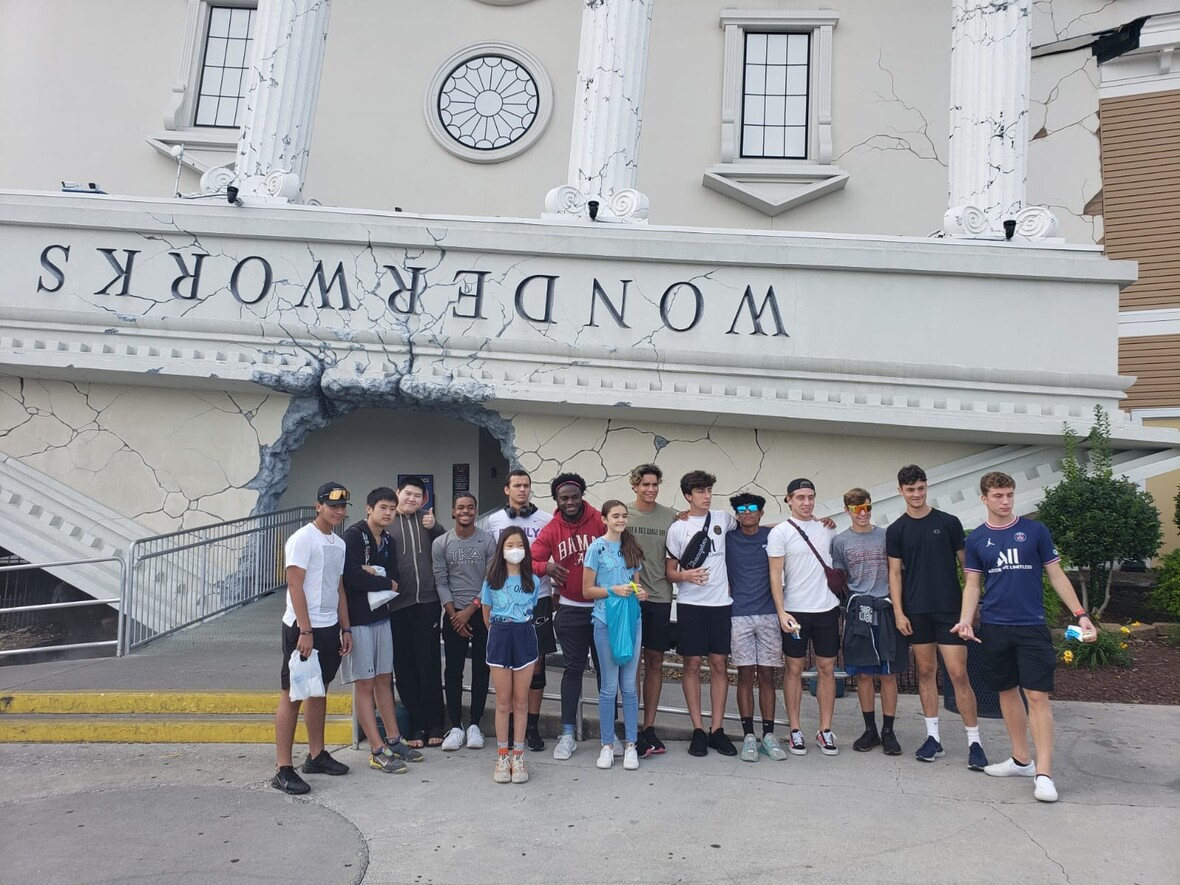# Residence Life in Action

This weekend, our residence life students spent time exploring the Pigeon Forge area! The first stop of the evening was WonderWorks! After spending time there, more excitement and adventures took place at the Nascar SpeedPark!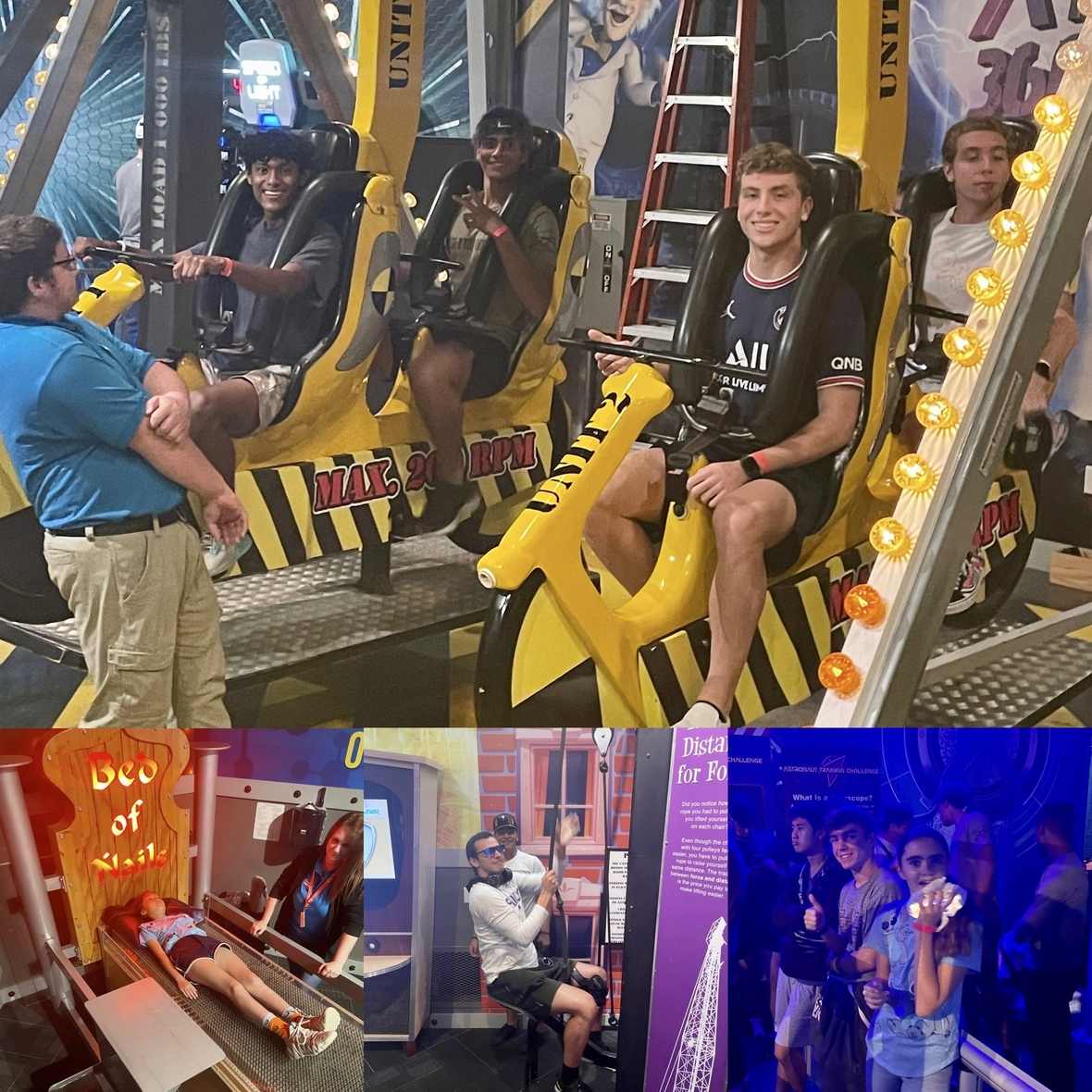table div table+table+table+table+table+table+table+table+table+table+table+table+table+table+table+table+table+table+table+table+table+table+table+table+table+table+table+table+table+table div table{width:100%;padding:0}table div table+table+table+table+table+table+table+table+table+table+table+table+table+table+table+table+table+table+table+table+table+table+table+table+table+table+table+table+table+table div table img{width:96.23%;padding:0;float:none}table div table+table+table+table+table+table+table+table+table+table+table+table+table+table+table+table+table+table+table+table+table+table+table+table+table+table+table+table+table+table div table td{width:100%;padding:0 1.88% 18px}/* styles */Check out the amazing academics happening on campus throughout our different grade levels!

Mrs. Polk and Mrs. Russell's have been learning about life cycles of plants and animals. Students had a day to "eat their science" as they categorized eggs, tadpoles, froglets, and frogs.

Then, the learning and fun continued as students created life cycle projects to present to their classmates!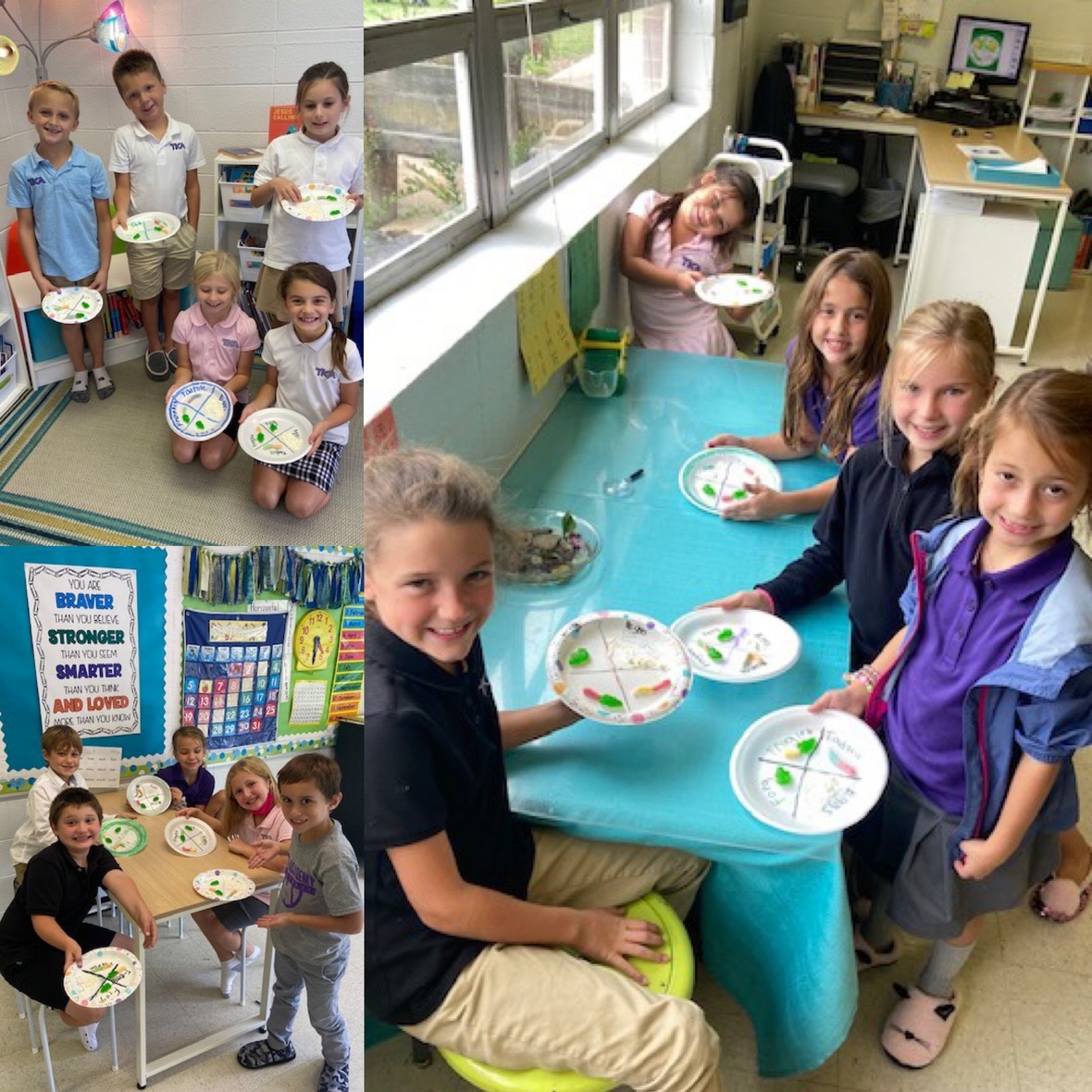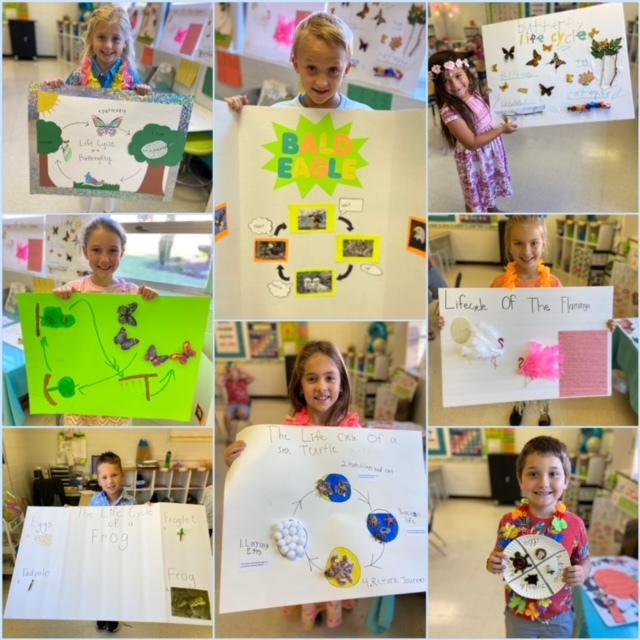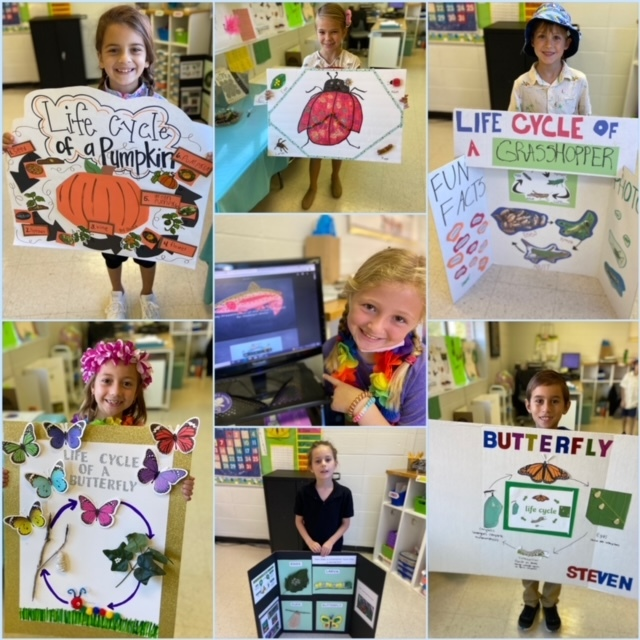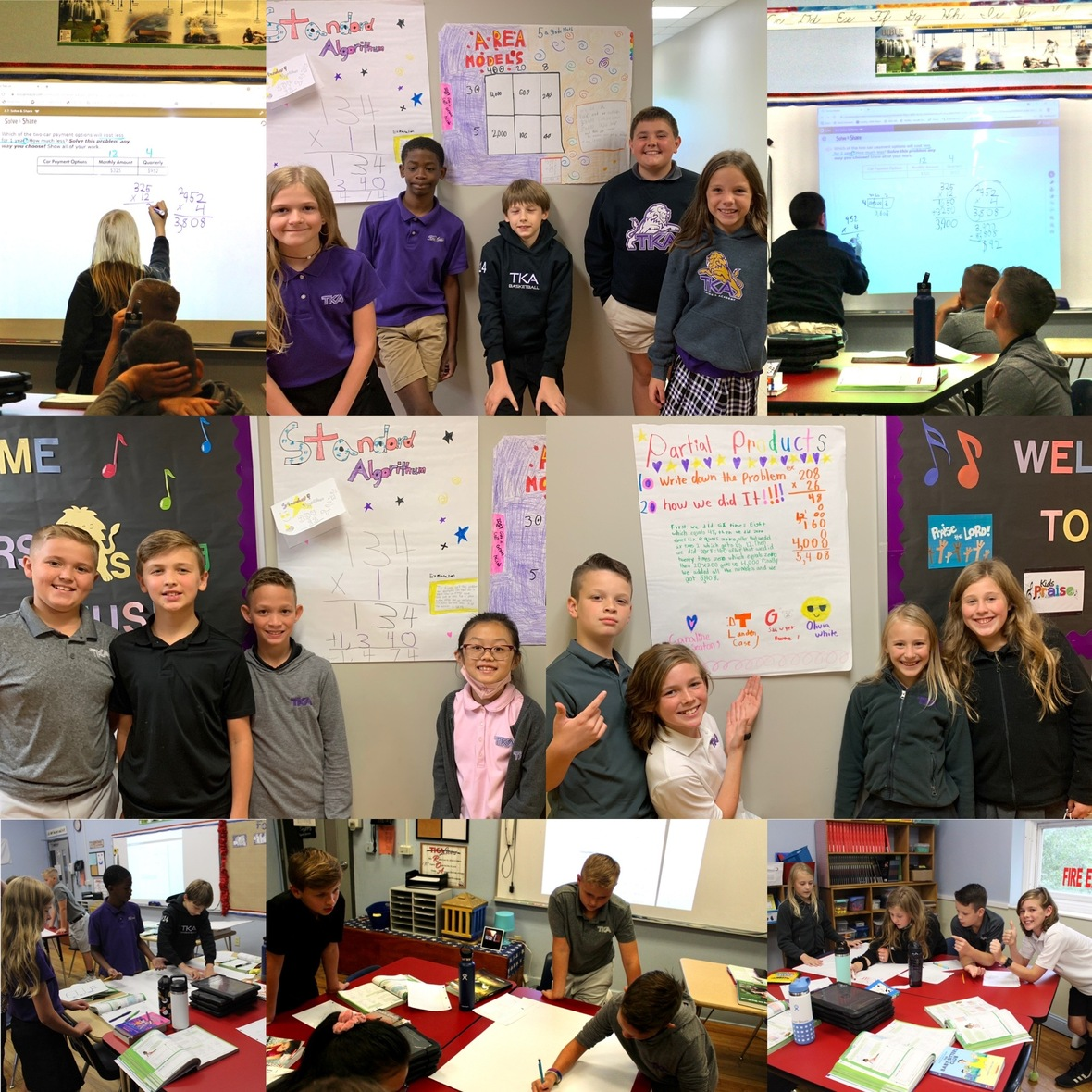Mrs. Quesinberry's 5th graders have been working on Standard Algorithm, Area Model, and Partial Product strategies! After learning these strategies, students worked together to solve problems. Once the problems were solved, each group took turns teaching their classmates how to use that way to solve the given problem.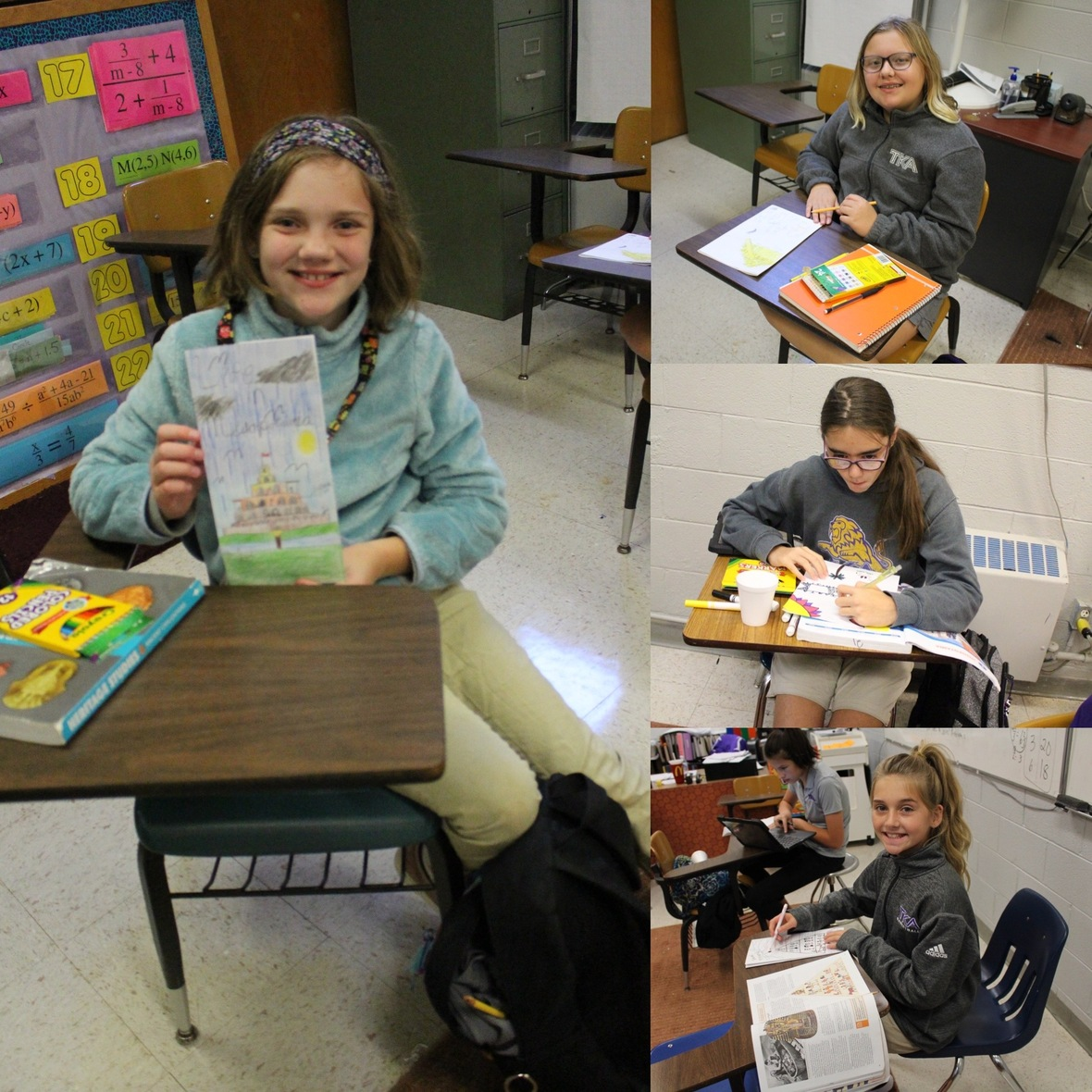Mrs. Sasscer's class has been learning about Mesopotamia. Students created their own book covers to highlighting some major accomplishments of this early civilization.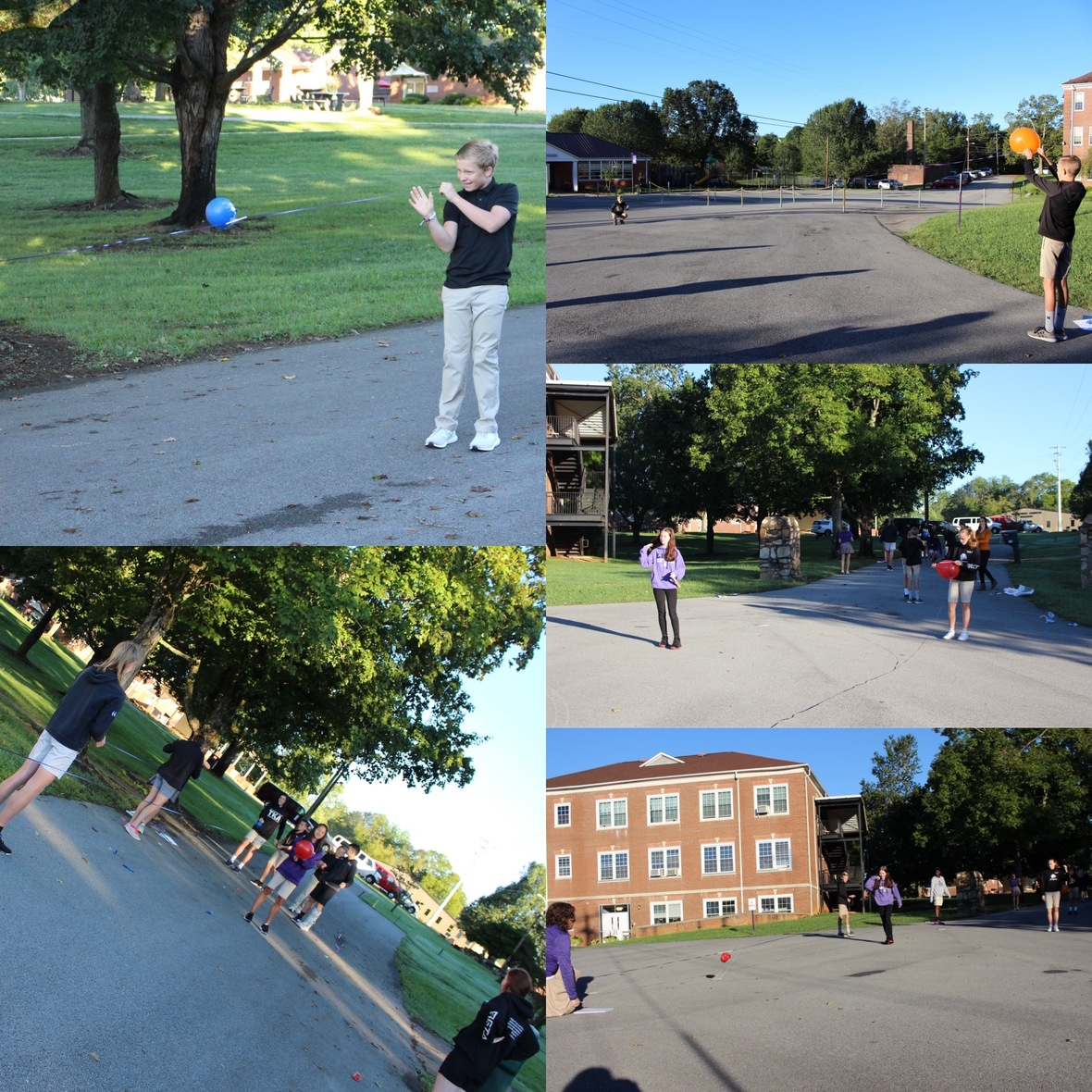Mrs. Neuhart's science class took their science experiments outside! Students demonstrated Newton's 3rd Law of Motion through rocket balloons!

After blowing up the balloons and letting it go down the string, students measured the distance traveled. Then, the groups made adjustments and completed the experiment again to see if the distance improved!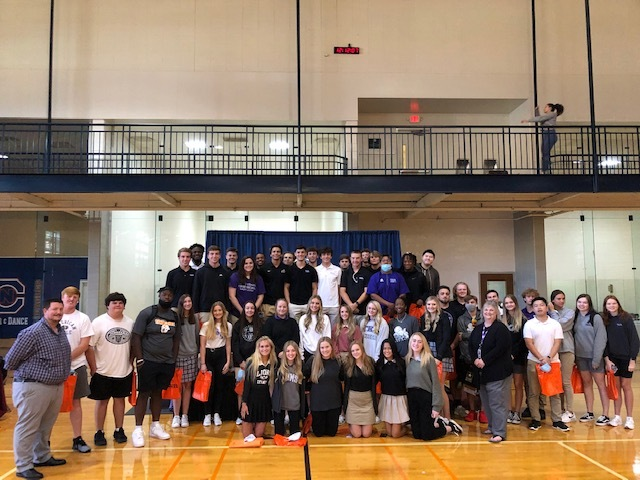## 11th and 12th Grade Students Visit Carson - Newman

This week Mrs. Hatmaker and Mr. Fuller took our Junior and Senior students on their first college visit of the school year!

Carson-Newman University hosted a college fair for students from around the area to view the opportunities provided by various institutions.

 table div table+table+table+table+table+table+table+table+table+table+table+table+table+table+table+table+table+table+table+table+table+table+table+table+table+table+table+table+table+table+table+table+table+table+table+table+table+table div table{width:100%;padding:0}table div table+table+table+table+table+table+table+table+table+table+table+table+table+table+table+table+table+table+table+table+table+table+table+table+table+table+table+table+table+table+table+table+table+table+table+table+table+table div table img{width:96.23%;padding:0;float:none}table div table+table+table+table+table+table+table+table+table+table+table+table+table+table+table+table+table+table+table+table+table+table+table+table+table+table+table+table+table+table+table+table+table+table+table+table+table+table div table td{width:100%;padding:0 1.88% 18px}/* styles */table div table+table+table+table+table+table+table+table+table+table+table+table+table+table+table+table+table+table+table+table+table+table+table+table+table+table+table+table+table+table+table+table+table+table+table+table+table+table+table+table div table{width:100%;padding:0}table div table+table+table+table+table+table+table+table+table+table+table+table+table+table+table+table+table+table+table+table+table+table+table+table+table+table+table+table+table+table+table+table+table+table+table+table+table+table+table+table div table img{width:96.23%;padding:0;float:none}table div table+table+table+table+table+table+table+table+table+table+table+table+table+table+table+table+table+table+table+table+table+table+table+table+table+table+table+table+table+table+table+table+table+table+table+table+table+table+table+table div table td{width:100%;padding:0 1.88% 18px}/* styles */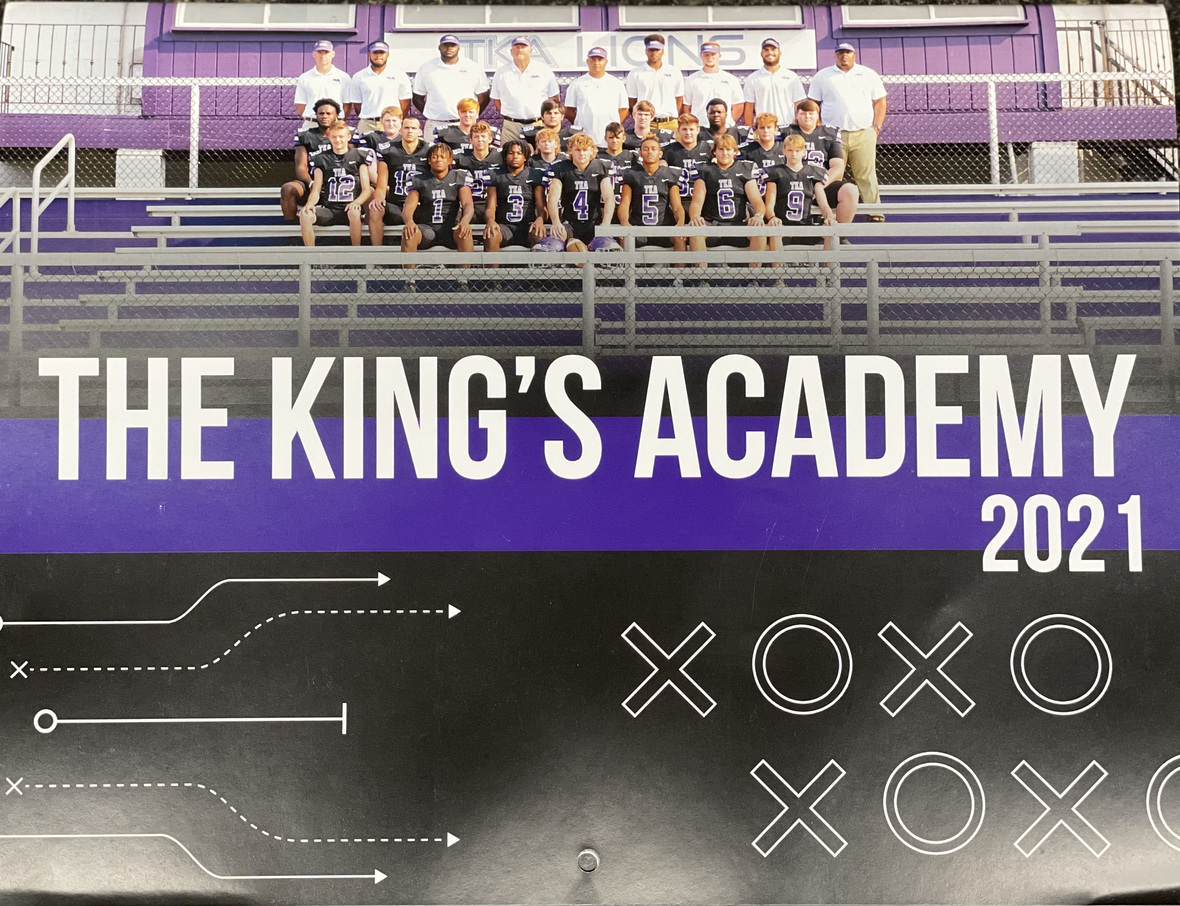# 2021-2022 Football Calendar

This year's football calendars are for sale for \$10! Stop by the school office to pick up your copy today!

 table div table+table+table+table+table+table+table+table+table+table+table+table+table+table+table+table+table+table+table+table+table+table+table+table+table+table+table+table+table+table+table+table+table+table+table+table+table+table+table+table+table+table div table{width:100%;padding:0}table div table+table+table+table+table+table+table+table+table+table+table+table+table+table+table+table+table+table+table+table+table+table+table+table+table+table+table+table+table+table+table+table+table+table+table+table+table+table+table+table+table+table div table img{width:96.23%;padding:0;float:none}table div table+table+table+table+table+table+table+table+table+table+table+table+table+table+table+table+table+table+table+table+table+table+table+table+table+table+table+table+table+table+table+table+table+table+table+table+table+table+table+table+table+table div table td{width:100%;padding:0 1.88% 18px}/* styles */table div table+table+table+table+table+table+table+table+table+table+table+table+table+table+table+table+table+table+table+table+table+table+table+table+table+table+table+table+table+table+table+table+table+table+table+table+table+table+table+table+table+table+table div table td,table.module-42{width:100%;padding:0}table div table+table+table+table+table+table+table+table+table+table+table+table+table+table+table+table+table+table+table+table+table+table+table+table+table+table+table+table+table+table+table+table+table+table+table+table+table+table+table+table+table+table+table div table{width:100%;float:none;margin-left:auto;margin-right:auto;padding:0}table div table+table+table+table+table+table+table+table+table+table+table+table+table+table+table+table+table+table+table+table+table+table+table+table+table+table+table+table+table+table+table+table+table+table+table+table+table+table+table+table+table+table+table div table a{border:0 none;text-decoration:none}table div table+table+table+table+table+table+table+table+table+table+table+table+table+table+table+table+table+table+table+table+table+table+table+table+table+table+table+table+table+table+table+table+table+table+table+table+table+table+table+table+table+table+table div table img{width:100%!important;border:0 none;text-decoration:none}/* styles */
 table div table+table+table+table+table+table+table+table+table+table+table+table+table+table+table+table+table+table+table+table+table+table+table+table+table+table+table+table+table+table+table+table+table+table+table+table+table+table+table+table+table+table+table+table div table{width:100%;padding:0}table div table+table+table+table+table+table+table+table+table+table+table+table+table+table+table+table+table+table+table+table+table+table+table+table+table+table+table+table+table+table+table+table+table+table+table+table+table+table+table+table+table+table+table+table div table img{width:96.23%;padding:0;float:none}table div table+table+table+table+table+table+table+table+table+table+table+table+table+table+table+table+table+table+table+table+table+table+table+table+table+table+table+table+table+table+table+table+table+table+table+table+table+table+table+table+table+table+table+table div table td{width:100%;padding:0 1.88% 18px}/* styles */# Hawaiian Dress up day recap!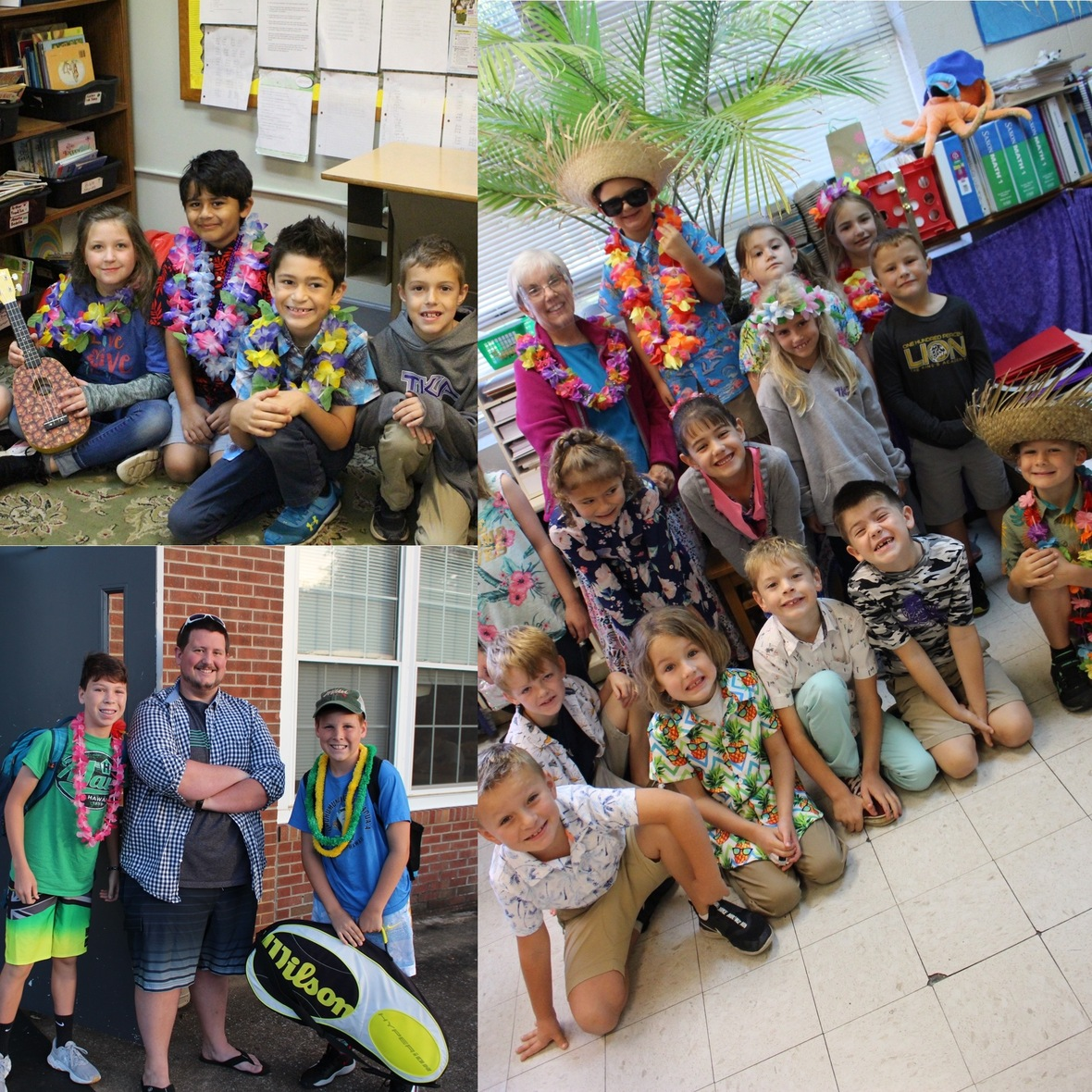table div table+table+table+table+table+table+table+table+table+table+table+table+table+table+table+table+table+table+table+table+table+table+table+table+table+table+table+table+table+table+table+table+table+table+table+table+table+table+table+table+table+table+table+table+table+table+table+table+table+table div table{width:100%;padding:0}table div table+table+table+table+table+table+table+table+table+table+table+table+table+table+table+table+table+table+table+table+table+table+table+table+table+table+table+table+table+table+table+table+table+table+table+table+table+table+table+table+table+table+table+table+table+table+table+table+table+table div table img{width:96.23%;padding:0;float:none}table div table+table+table+table+table+table+table+table+table+table+table+table+table+table+table+table+table+table+table+table+table+table+table+table+table+table+table+table+table+table+table+table+table+table+table+table+table+table+table+table+table+table+table+table+table+table+table+table+table+table div table td{width:100%;padding:0 1.88% 18px}/* styles */# Covid-19 Procedures

While we are excited to offer in-person education, we must continue to maintain a safe learning environment for all students, faculty/staff, and additional stakeholders.

To do so, we will be working closely with the Sevier County Health Department in regards to Covid-19 protocols.

 table div table+table+table+table+table+table+table+table+table+table+table+table+table+table+table+table+table+table+table+table+table+table+table+table+table+table+table+table+table+table+table+table+table+table+table+table+table+table+table+table+table+table+table+table+table+table+table+table+table+table+table+table div table{width:100%;padding:0}table div table+table+table+table+table+table+table+table+table+table+table+table+table+table+table+table+table+table+table+table+table+table+table+table+table+table+table+table+table+table+table+table+table+table+table+table+table+table+table+table+table+table+table+table+table+table+table+table+table+table+table+table div table img{width:96.23%;padding:0;float:none}table div table+table+table+table+table+table+table+table+table+table+table+table+table+table+table+table+table+table+table+table+table+table+table+table+table+table+table+table+table+table+table+table+table+table+table+table+table+table+table+table+table+table+table+table+table+table+table+table+table+table+table+table div table td{width:100%;padding:0 1.88% 18px}/* styles */table div table+table+table+table+table+table+table+table+table+table+table+table+table+table+table+table+table+table+table+table+table+table+table+table+table+table+table+table+table+table+table+table+table+table+table+table+table+table+table+table+table+table+table+table+table+table+table+table+table+table+table+table+table+table div table{width:100%;padding:0}table div table+table+table+table+table+table+table+table+table+table+table+table+table+table+table+table+table+table+table+table+table+table+table+table+table+table+table+table+table+table+table+table+table+table+table+table+table+table+table+table+table+table+table+table+table+table+table+table+table+table+table+table+table+table div table img{width:96.23%;padding:0;float:none}table div table+table+table+table+table+table+table+table+table+table+table+table+table+table+table+table+table+table+table+table+table+table+table+table+table+table+table+table+table+table+table+table+table+table+table+table+table+table+table+table+table+table+table+table+table+table+table+table+table+table+table+table+table+table div table td{width:100%;padding:0 1.88% 18px}/* styles */